# Milo lifted

Milo lifted 65 lb 6 times and Jeremy lifted 50 lb 9 times. Which one lifted the most weight?

Result

w = (Correct answer is: J)#### Solution:Leave us a comment of example and its solution (i.e. if it is still somewhat unclear...):

Showing 0 comments:Be the first to comment!## Next similar examples:

1. SickSick Marcel already taken six tablets, which was a quarter of the total number of pills in the pack. How many pills were in the pack?
2. Bread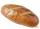5 of the same bread has the same weight as three bread and 4 kg of fruit. What weight has one bread?
3. Solid food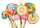Stacie is a resident at the medical facility where you work. You are asked to chart the amount of solid food that she consumes. For the noon meal, today she ate 1/2 of a 3-ounce serving of meatloaf, 3/4 of her 3-ounce serving of mashed potatoes, and 1/3 of
4. Coal mine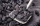The monthly plan of 17,000 tons of coal exceeded the mine by 1/25. How many tonnes of coal have been harvested from the mine above plan?
5. Pound2kilosHow many pounds make 1 kilograms?
6. RecipeA recipe requires 2 pounds of flour. If a chef wants to triple the recipe, how many ounces of flour will be needed?
7. Without 2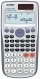Without multipying, tell whether the product 0.644 x 0.25 will be greater than 1 or less than 1? Explane how you know. Then find the product.
8. Equivalent fractionsAre these two fractions equivalent -4/9 and 11/15?
9. Compare: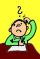What is bigger? ?
10. MidpointsTriangle whose sides are midpoints of sides of triangle ABC has a perimeter 45. How long is perimeter of triangle ABC?
11. Negative in equation2x + 3 + 7x = – 24, what is the value of x?
12. Cupcakes 2Susi has 25 cupcakes. She gives 4/5. How much does she have left?
13. Find xSolve: if 2(x-1)=14, then x= (solve an equation with one unknown)
14. TeacherTeacher Rem bought 360 pieces of cupcakes for the outreach program of their school. 5/9 of the cupcakes were chocolate flavor and 1/4 wete pandan flavor and the rest were vanilla flavor. How much more chocolate flavor cupcakes than vanilla flavor?
15. DisjointHow many elements have union and intersection of two disjoint sets when the first have 1 and secodn 8 elements.
16. Simple equationSolve the following simple equation: 2. (4x + 3) = 2-5. (1-x)
17. Fractions 3Calculate 1/9 of 27: